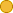## ← Mean Lifetime - Differential Equations in Action

• 2 Followers
• 34 Lines

### Get Embed Code x Embed video Use the following code to embed this video. See our usage guide for more details on embedding. Paste this in your document somewhere (closest to the closing body tag is preferable): ```<script type="text/javascript" src='https://amara.org/embedder-iframe'></script> ``` Paste this inside your HTML body, where you want to include the widget: ```<div class="amara-embed" data-url="http://www.youtube.com/watch?v=iufHI-HZ9Pg" data-team="udacity"></div> ``` 3 Languages

•English [en] original
•Spanish [es]
•Russian [ru] incomplete

Showing Revision 2 created 05/25/2016 by Udacity Robot.

1. Now let's try to apply the same idea to the exponential decay.
2. You start with some initial number of particles--N of zero seconds--and decay smoothly with that exponential curve.
3. If we have this area below that curve, it's easy to say that the mean lifetime equals that area
4. divided by the initial number of particles. Here comes a trick:
5. I introduce a different area. I start at a time H, close to zero. And look at this area.
6. Let's call that A of H, and consequently call this A of zero seconds.
7. The funny thing about A of H is that it's a scaled down version of that original red area.
8. If you multiply with the right factor, you're going to turn that red area into that green area.
9. You might multiply with the right number to get this curve from the blue curve,
10. and the area below that curve equals that green area.
11. The right factor is easy to figure out. It's the ratio of the number of particles present at time H
12. to the number of particles present initially.
13. So this is N of H over N of zero seconds.
14. For H close to zero, we can use the differential equation to get an estimate on N of H, the number of particles
15. present at time H. That's the initial number minus how many particles have decayed.
16. If you use the rate of change, then it's one over 20 seconds times the initial number, times H,
17. the time that we waited. That's, of course, just an estimate.
18. If I want this to be more accurate, I need to take care of the remainder, which is something of order H squared.
19. And now here's the tiny area left. This area amounts to its width, H, times its height, the initial number of particles,
20. where not perfectly, we're neglecting something, but that's of order H squared.
21. And now let's put all of that together. The complete area--the red area--equals the blue area plus the green area.
22. So that's the initial number of particles minus one over 20 seconds times the initial number of particles,
23. times H, plus something of the order of H squared, divided by the initial number of particles,
24. times the total area. And now look at what remains: We've got the complete area on the left-hand side,
25. and we've got the initial number divided by the initial number, times the complete area on the right-hand side.
26. We can get rid of these. Then we have terms that are linear in H, then we have terms that are of first order in H,
27. H times a constant, H times a constant, and we have terms that are of second order in H.
28. The first order terms have to match, and the second order terms have to match.
29. So we can get rid of the second order terms. Now we can cancel the initial number here
30. and the initial number there, and you see that zero equals H times the initial number, minus one over 20 seconds
31. times H, times the complete area. Now we can also get rid of H, to find that the area divided by the initial number
32. equals 20 seconds. So this ratio, which is the mean lifetime, equals, guess what? 20 seconds.
33. So astonishingly, this number that appears in the differential equation is nothing but the mean lifetime.
34. It's that easy.